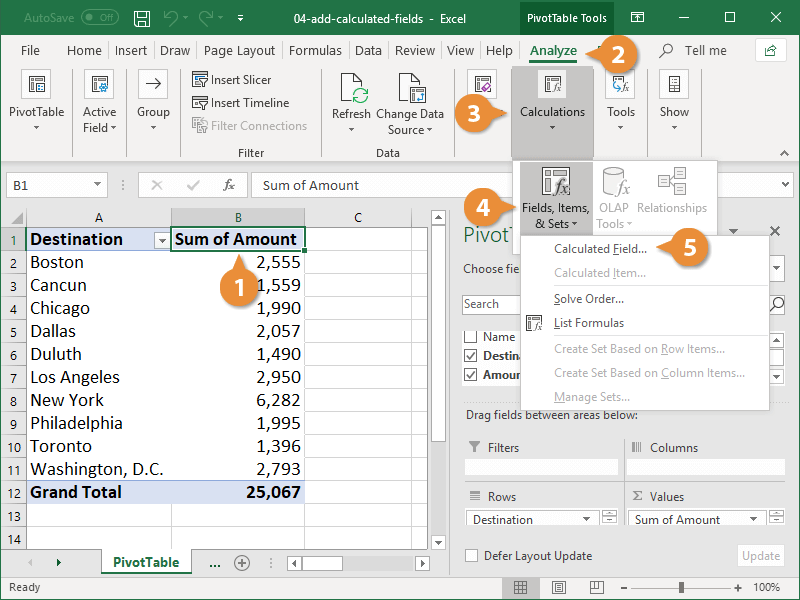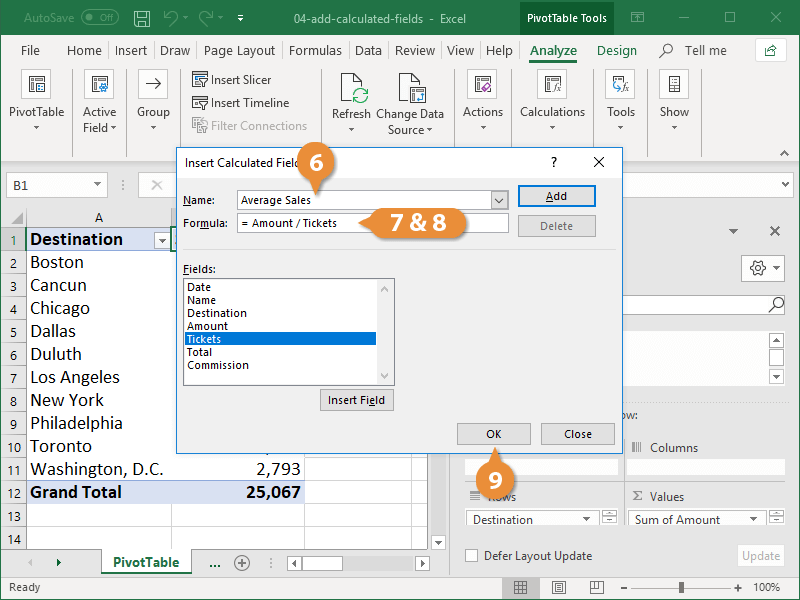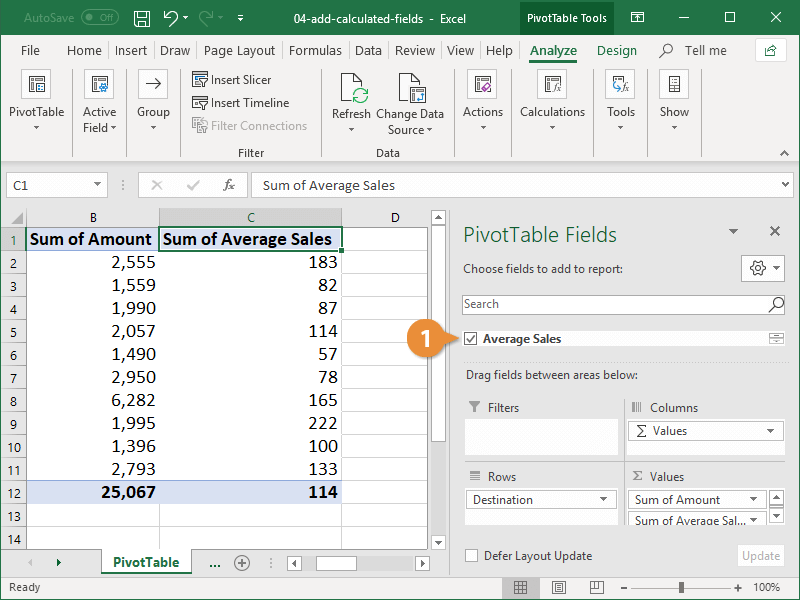Pivot Table Calculated Field | CustomGuide# Pivot Table Calculated Field

How to Add and Remove Calculated Fields in Excel PivotTablesA calculated field is a new field that performs calculations based on existing fields in your PivotTable. For example, you could use a calculated field to find the average ticket sales using an existing total sales field and a field containing the number of tickets sold.

### Create a Calculated Field

1. Click a cell in the PivotTable.
2. Click the Analyze tab.
3. Expand the Calculations group, if necessary.
4. Click the Fields, Items, & Sets button.
5. Select Calculated Field.The Insert Calculated Field dialog box displays. This is where you’ll create and name the field.

6. Type a name for the calculated field in the Name field.
7. Click in the Formula field.
8. Enter a calculation using the fields in the list and/or custom values.

You can also enter your own values in the formula. For example, you could find a monthly average by adding an existing field and then dividing by 12.

9. Click OK.
10.The new calculated field is automatically added to the PivotTable.

### Remove a Calculated Field

If you decide you don't need the calculated field in your PivotTable, it can be removed just like any other PivotTable field.

1. Uncheck the calculated field in the PivotTable Fields pane.The calculated field is removed from the PivotTable; however, it remains in the field list to use again in the future.

If you want to remove the calculated field completely, you need to re-open the Insert Calculated Field dialog box, select the field you created in the Fields list, and click the Delete button.